Example Questions

Example Question #2 : How To Find The Common Difference In Sequences

In a sequence of numbers, each term istimes larger than the one before it. If the 3rd term of the sequence is 12, and the 6th term is 96, what is the sum of all of the terms less than 250?

381

372

378

192

384

381

Explanation:

Let's call the first term in the sequence a1 and the nth term an

We are told that each term is r times larger than the one before it. Thus, we can find the next term in the sequence by multiplying by r.

a1 = a1

a2 = r(a1)

a3 = r(a2) = r(r(a1)) = r2(a1)

a4 = r(a3) = r(r2(a1)) = r3(a1)

an = r(n–1)a1

We can use this information to find r.

The problem gives us the value of the third and the sixth terms.

a3 = r2(a1) = 12

a6 = r5(a1) = 96

Let's solve for a1 in terms of r and a3

a1 = 12/(r2)

Let's then solve for a1 in terms of r and a6.

a1 = 96/(r5)

Now, we can set both values equal and solve for r.

12/(r2) = 96/(r5)

Multiply both sides by r5 to get rid of the fraction.

12r5/r2 = 96

Apply the property of exponents which states that ab/ac = ab–c.

12r3 = 96

Divide by 12 on both sides.

r3 = 8

Take the cube root of both sides.

r = 2

This means that each term is two times larger than the one before it, or that each term is one half as large as the one after it.

a2 must equal a3 divided by 2, which equals 12/2 = 6.

a1 must equal a2 divided by 2, which equals 6/2 = 3.

Here are the first eight terms of the sequence:

3, 6, 12, 24, 48, 96, 192, 384

The question asks us to find the sum of all the terms less than 250. Only the first seven terms are less than 250. Thus the sum is equal to the following:

sum = 3 + 6 + 12 + 24 + 48 + 96 + 192 = 381

Example Question #11 : Sequences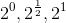Find the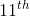term of the sequence above.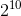Explanation:

The sequence is geometric with a common ratio of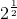.

The formula for finding the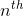term of the sequence is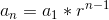.

So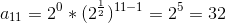Example Question #6 : How To Find The Common Difference In Sequences

Which of the following are not natural numbers?

I. 1

II. 0

III. 349010

IV. -2

V. 1/4

I, V

IV, V

II, IV, V

II, III, IV, V

I, IV, V

II, IV, V

Explanation:

Natural numbers are defined as whole numbers 1 and above. II, IV, V are not natural numbers.

Example Question #12 : Sequences

An arithmetic sequence begins as follows: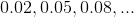Give the first integer in the sequence.The sequence has no integers.Explanation:

Subtract the first term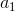from the second term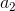to get the common difference: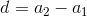Setting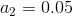and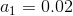,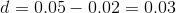Ifis in the sequence, then there is an integersuch that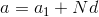, or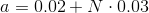Solving for,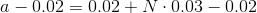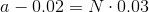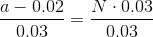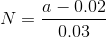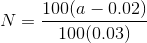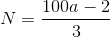Therefore, we seek the least positive integer value ofsuch thatis itself an integer. By trial and error, we see: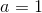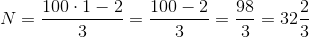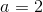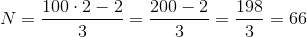,

which is an integer.

Therefore, 2 is the first integer value in the sequence.

Example Question #1 : Arithmetic Sequences

-27, -24, -21, -18…

In the sequence above, each term after the first is 3 greater than the preceding term. Which of the following could not be a value in the sequence?

657

501

461

126

461

Explanation:

All of the values in the sequence must be a multiple of 3. All answers are multiples of 3 except 461 so 461 cannot be part of the sequence.

Example Question #2 : Arithmetic Sequences

m, 3m, 5m, ...

The first term in the above sequence is m, and each subsequent term is equal to 2m + the previous term. If is an integer, then which of the following could NOT be the sum of the first four terms in this sequence?

80

48

60

16

-32

60

Explanation:

The fourth term of this sequence will be 5m + 2m = 7m. If we add up the first four terms, we get m + 3m + 5m + 7m = 4m + 12m = 16m. Since m is an integer, the sum of the first four terms, 16m, will have a factor of 16. Looking at the answer choices, 60 is the only answer where 16 is not a factor, so that is the correct choice.

Example Question #3 : Arithmetic Sequences

The tenth term in a sequence is 40, and the twentieth term is 20. The difference between consequence terms in the sequence is constant. Find n such that the sum of the first n numbers in the sequence equals zero.

59
40
30
58
60
Explanation:

Let d represent the common difference between consecutive terms.

Let an denote the nth term in the sequence.

In order to get from the tenth term to the twentieth term in the sequence, we must add d ten times.

Thus a20 = a10 + 10d

20 = 40 + 10d

d = -2

In order to get from the first term to the tenth term, we must add d nine times.

Thus a10 = a1 + 9d

40 = a1 + 9(-2)

The first term of the sequence must be 58.

Our sequence looks like this: 58,56,54,52,50…

We are asked to find the nth term such that the sum of the first n numbers in the sequence equals 0.

58 + 56 + 54 + …. an = 0

Eventually our sequence will reach zero, after which the terms will become the negative values of previous terms in the sequence.

58 + 56 + 54 + … 6 + 4 + 2 + 0 + -2 + -4 + -6 +….-54 + -56 + -58 = 0

The sum of the term that equals -2 and the term that equals 2 will be zero. The sum of the term that equals -4 and the term that equals 4 will also be zero, and so on.

So, once we add -58 to all of the previous numbers that have been added before, all of the positive terms will cancel, and we will have a sum of zero. Thus, we need to find what number -58 is in our sequence.

It is helpful to remember that a= a+ d(n-1), because we must add d to aexactly n-1 times in order to give us an. For example, a5 = a1 + 4d, because if we add d four times to the first term, we will get the fifth term. We can use this formula to find n.

-58 = an = a1 + d(n-1)

-58 = 58 + (-2)(n-1)

n = 59

Example Question #4 : Arithmetic Sequences

The first term of a sequence is 1, and every term after the first term is –2 times the preceding term. How many of the first 50 terms of this sequence are less than 5?

8

64

16

27

54

27

Explanation:

We can see how the sequence begins by writing out the first few terms:

1, –2, 4, –8, 16, –32, 64, –128.

Notice that every other term (of which there are exactly 50/2 = 25) is negative and therefore less than 25. Also notice that after the fourth term, every term is greater in absolute value than 5, so we just have to find the number of positive terms before the fourth term that are less than 5 and add that number to 25 (the number of negative terms in the first 50 terms).

Of the first four terms, there are only two that are less than 5 (i.e. 1 and 4), so we include these two numbers in our count: 25 negative numbers plus an additional 2 positive numbers are less than 5, so 27 of the first 50 terms of the sequence are less than 5.

Example Question #5 : Arithmetic Sequences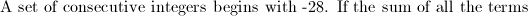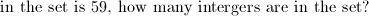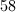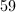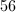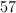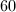Explanation:

Look for cancellations to simplify.  The sum of all consecutive integers from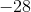tois equal to. Therefore, we must go a little farther.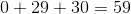, so the last number in the sequence in.  That gives usnegative integers,positive integers, and don't forget zero!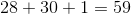.

Example Question #6 : Arithmetic Sequences

Brad can walk 3600 feet in 10 minutes. How many yards can he walk in ten seconds?